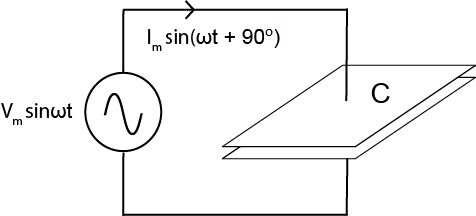# Capacitor Impedance Calculator

## This tool calculates a capacitor's reactance for a given capacitance value and signal frequency.

### Overview

Our capacitive reactance calculator helps you determine the impedance of a capacitor if its capacitance value (C) and the frequency of the signal passing through it (f) are given. You can input the capacitance in farads, microfarads, nanofarads, or picofarads. For the frequency, the unit options are Hz, kHz, MHz, and GHz.### Equation

$$X_{C} = \frac{1}{\omega C} = \frac{1}{2 \pi fC}$$

Where:

$$X_{C}$$ = capacitor reactance in ohms (Ω)

$$\omega$$ = angular frequency in rad/s = $$2 \pi f$$, where $$f$$ is the frequency in Hz

$$C$$ = capacitance in farads

Reactance (X) conveys a component's resistance to alternating current. Impedance (Z) conveys a component's resistance to both direct current and alternating current; it is expressed as a complex number, i.e., Z = R + jX. The impedance of an ideal resistor is equal to its resistance; in this case, the real part of the impedance is the resistance, and the imaginary part is zero. The impedance of an ideal capacitor is equal in magnitude to its reactance, but these two quantities are not identical. Reactance is expressed as an ordinary number with the unit ohms, whereas the impedance of a capacitor is the reactance multiplied by -j, i.e., Z = -jX. The -j term accounts for the 90-degree phase shift between voltage and current that occurs in a purely capacitive circuit.

The above equation gives you the reactance of a capacitor. To convert this to the impedance of a capacitor, simply use the formula Z = -jX. Reactance is a more straightforward value; it tells you how much resistance a capacitor will have at a certain frequency. Impedance, however, is needed for comprehensive AC circuit analysis.

As you can see from the above equation, a capacitor's reactance is inversely proportional to both frequency and capacitance: higher frequency and higher capacitance both lead to lower reactance. The inverse relationship between reactance and frequency explains why we use capacitors to block low-frequency components of a signal while allowing high-frequency components to pass.

Textbook - AC Capacitor Circuits

Textbook - Series Resistor-Capacitor Circuits

Worksheet - Capacitive Reactance

Clean Power for Every IC: Understanding Bypass Capacitors• W
WA7PRC September 02, 2016

Since it calculates only Xc (which is NOT impedance), this is not a very useful tool. Who DOESN’T recall the formula for Xc?

Frequency can be entered in ONLY Hertz, making this tool not very user-friendly above audio frequencies. Those of us working into the GHz region would have to enter LOTS of zeros.

Like.
• R
robertjt April 04, 2018
Impedance is the total resistance in ohms of any network at a specific frequency including both the real and imaginary of angular parts. Capacitors have a resistance that is totally imaginary with a vector of 90 degrees. The current lags the voltage by 90 degrees. An inductor is negative 90 degrees. Impedence includes all the resistances in a network along with the total phase shift introduced by all the components
Like.
•DDempsey February 27, 2023
What extra would you want in the the tool? Many of us haven't looked at it for decades - that's why I've checked my memory for a student. As for forumulas specifically for various frequency ranges with a scale factor out front.... that's a hangover from Imperial units where part of it is a scale factor and part is a unit transformation. MKS units are consistent and have consistent dimensions. In any case, that's what scientific / engineering notation is for: Xc = 1/(2.Pi. 9x10^9 . 1x10-6) is reactance of a 1 uF at 9 GHz. Your calculator can handle it. (1E9 is 1 G)
Like.